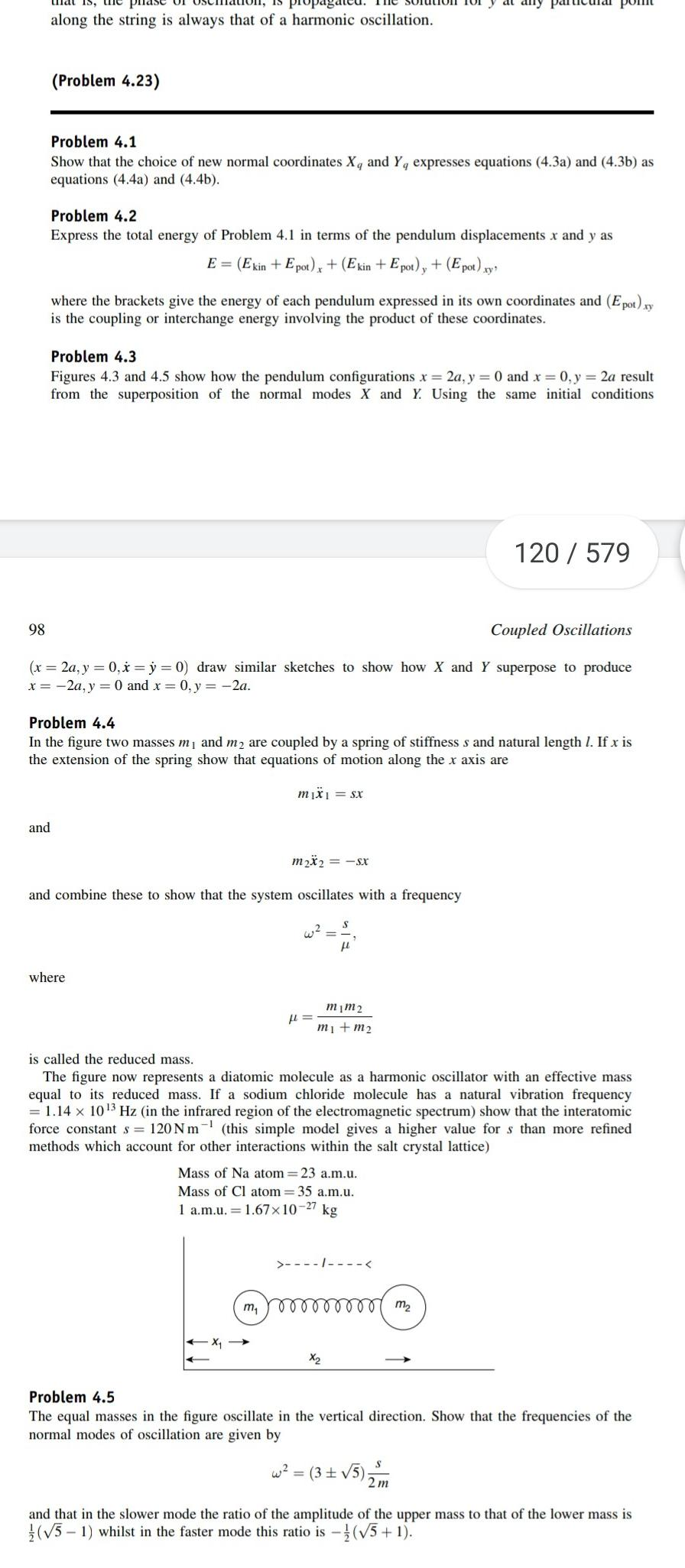Question:

# 98 along the string is always that of a harmonic oscillation

Last updated: 6/9/202398 along the string is always that of a harmonic oscillation Problem 4 23 Problem 4 1 Show that the choice of new normal coordinates X and Y expresses equations 4 3a and 4 3b as equations 4 4a and 4 4b Problem 4 2 Express the total energy of Problem 4 1 in terms of the pendulum displacements x and y as E Ekin Epot x Ekin Epot Epot xy where the brackets give the energy of each pendulum expressed in its own coordinates and Epot xy is the coupling or interchange energy involving the product of these coordinates Problem 4 3 Figures 4 3 and 4 5 show how the pendulum configurations x 2a y 0 and x 0 y 2a result from the superposition of the normal modes X and Y Using the same initial conditions and Coupled Oscillations x 2a y 0 x y 0 draw similar sketches to show how X and Y superpose to produce x 2a y 0 and x 0 y 2a Problem 4 4 In the figure two masses m and m2 are coupled by a spring of stiffness s and natural length 1 If x is the extension of the spring show that equations of motion along the x axis are m x1 Sx m x 2 sx and combine these to show that the system oscillates with a frequency where w X l m S fl m1m2 m m Mass of Na atom 23 a m u Mass of Cl atom 35 a m u 1 a m u 1 67 10 27 kg y is called the reduced mass The figure now represents a diatomic molecule as a harmonic oscillator with an effective mass equal to its reduced mass If a sodium chloride molecule has a natural vibration frequency 1 14 x 10 3 Hz in the infrared region of the electromagnetic spectrum show that the interatomic force constant s 120 Nm this simple model gives a higher value for s than more refined methods which account for other interactions within the salt crystal lattice mmmmmm m X 120 579 S 2m Problem 4 5 The equal masses in the figure oscillate in the vertical direction Show that the frequencies of the normal modes of oscillation are given by w 3 5 and that in the slower mode the ratio of the amplitude of the upper mass to that of the lower mass is 5 1 whilst in the faster mode this ratio is 5 1